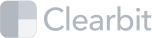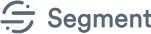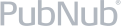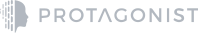# The Beginner’s Guide to Text Vectorization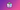Since the beginning of the brief history of Natural Language Processing (NLP), there has been the need to transform text into something a machine can understand. That is, transforming text into a meaningful vector (or array) of numbers. The de-facto standard way of doing this in the pre-deep learning era was to use a bag of words approach.

## Bag of words

The idea behind this method is straightforward, though very powerful. First, we define a fixed length vector where each entry corresponds to a word in our pre-defined dictionary of words. The size of the vector equals the size of the dictionary. Then, for representing a text using this vector, we count how many times each word of our dictionary appears in the text and we put this number in the corresponding vector entry.

For example, if our dictionary contains the words {MonkeyLearn, is, the, not, great}, and we want to vectorize the text “MonkeyLearn is great”, we would have the following vector: (1, 1, 0, 0, 1).

To improve this representation, you can use some more advanced techniques like removing stopwords, lemmatizing words, using n-grams or using tf-idf instead of counts.

The problem with this method is that it doesn’t capture the meaning of the text, or the context in which words appear, even when using n-grams.

## Deep Learning is transforming text vectorization

Since deep learning has taken over the machine learning field, there have been many attempts to change the way text vectorization is done and find better ways to represent text.

One of the first steps that were taken to solve this problem was to find a way to vectorize words, which became very popular with the word2vec implementation back in 2013. By using vast amounts of data, it is possible to have a neural network learn good vector representations of words that have some desirable properties like being able to do math with them. For example, with word2vec you can do “king” - “man” + “woman” and you get as a result a vector that is very similar to the vector “queen”. This actually seems like magic, I recommend reading this blogpost if you are interested in knowing how this is possible. These vectors are useful for doing a lot of tasks related to NLP because each of its dimensions encode a different property of the word. For example, you can have one property that describes if the word is a verb or a noun, or if the word is plural or not.

The next step is to get a vectorization for a whole sentence instead of just a single word, which is very useful if you want to do text classification for example. This problem has not been completely addressed yet, but there have been some significant advances in the last few years with implementations like the Skip-Thought Vectors.

## Transfer Learning

In the field of machine learning, transfer learning is the ability of the machine to use some of the learned concepts in one task for another different task. One of the problems of the bag of words approach for text vectorization is that for each new problem that you face, you need to do all the vectorization from scratch.

Humans don’t have this problem; we know that certain words have particular meanings, and we know that these meanings may change in different contexts. We don’t need to learn again the meaning of the words each time we read a book.

One of the promises in the deep learning field is the ability to have text vectorizations that can be used in multiple different problems, and don’t have to be learned over and over again.

## Skip-Thought Vectors

Multiple research is being done in this direction and one of the best results found yet are the Skip-Thoughts vectors developed by the University of Toronto. An implementation of this algorithm using Theano can be found here.

The idea behind this algorithm is the following: in the same way we can get a good word vector representation by using a neural network that tries to predict the surrounding words of a word, they use a neural network to predict the surrounding sentences of a sentence. For this to work, they needed huge amounts of contiguous text data, which they found in the BookCorpus dataset. These are free books written by yet unpublished authors.

In their paper, they demonstrate that these sentence vectors can be used as a very robust text representation. We are going to try this out in a text classification problem, to see if it is worth to use it in a real-world problem.

## A practical example

We are going to use Scikit-Learn and the skip-thoughts implementation (that uses Theano) given in the paper, to compare Skip-Thoughts vs Bag of Words approach. We are going to use the Airline Sentiment dataset, composed of 16,000 tweets that talk about airlines and has three classes: Positive, Negative and Neutral. We will use accuracy as our metric to evaluate the classification results.

First, we need to load the data. We split the dataset in two, train and test:

``````import csv
import random

f = open('train.csv')
train_rows = [row for row in csv.reader(f)][1:]  # discard the first row
random.shuffle(train_rows)
tweets_train = [row.decode('utf8') for row in train_rows]
classes_train = [row for row in train_rows]

f = open('test.csv')
test_rows = [row for row in csv.reader(f)][1:]  # discard the first row
tweets_test = [row.decode('utf8') for row in test_rows]
classes_test = [row for row in test_rows]
``````

Now we will define a vectorizer class that we will later use in a scikit-learn pipeline:

``````import skipthoughts

class SkipThoughtsVectorizer(object):
def __init__(self, **kwargs):
self.encoder = skipthoughts.Encoder(self.model)

def fit_transform(self, raw_documents, y):
return self.encoder.encode(raw_documents, verbose=False)

def fit(self, raw_documents, y=None):
self.fit_transform(raw_documents, y)
return self

def transform(self, raw_documents, copy=True):
return self.fit_transform(raw_documents, None)
``````

Now we will define three scikit-learn pipelines. One that uses our SkipThoughtsVectorizer, other that uses the TF-IDF bag of n-grams approach, and a third one that uses a combination of both. In the three of them, we will use a simple Logistic Regression model for classifying our data.

``````from sklearn.pipeline import Pipeline, FeatureUnion
from sklearn.linear_model import LogisticRegression
from sklearn.feature_extraction.text import TfidfVectorizer

pipeline_skipthought = Pipeline(steps=[('vectorizer', SkipThoughtsVectorizer()),
('classifier', LogisticRegression())])
pipeline_tfidf = Pipeline(steps=[('vectorizer', TfidfVectorizer(ngram_range=(1, 2))),
('classifier', LogisticRegression())])

feature_union = ('feature_union', FeatureUnion([
('skipthought', SkipThoughtsVectorizer()),
('tfidf', TfidfVectorizer(ngram_range=(1, 2))),
]))
pipeline_both = Pipeline(steps=[feature_union,
('classifier', LogisticRegression())])
``````

Finally, we will train these 3 pipelines with our train split and test with the test split. We want to see how accuracy grows the more data we feed to the algorithm. So we will train the models many times with different training dataset sizes, but always testing with the whole test split:

``````for train_size in (20, 50, 100, 200, 500, 1000, 2000, 3000, len(tweets_train)):
print(train_size, '--------------------------------------')
# skipthought
pipeline_skipthought.fit(tweets_train[:train_size], classes_train[:train_size])
print ('skipthought', pipeline_skipthought.score(tweets_test, classes_test))

# tfidf
pipeline_tfidf.fit(tweets_train[:train_size], classes_train[:train_size])
print('tfidf', pipeline_tfidf.score(tweets_test, classes_test))

# both
pipeline_both.fit(tweets_train[:train_size], classes_train[:train_size])
print('skipthought+tfidf', pipeline_both.score(tweets_test, classes_test))
``````

Here we can see a graph of how accuracy grows when feeding the models with more data: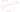You can get the full code to replicate these results here.

### Results

When having little data to train (from 0 to 5000 texts), the Skip-Thoughts approach worked better than the Bag of n-grams. This tell us that we were effectively able to use some transfer learning in this task to improve our results. Since the Skip-Thoughts approach had already some information about words meaning, it was able to use this information to provide better classification results.

On the other hand, when given the full training data, the Bag of n-grams outperformed the other approach. We may conclude with this that while having some information about what words mean can be useful to do sentiment classification, it was more useful to have specific information about the dataset itself. The Bag of n-grams approach was able to better “understand” this dataset-specific information.

When combining the two approaches, we can see that we have a boost in the accuracy of our model. This means that Skip-Thoughts and Bag of n-grams hold complementary information about the training set. Skip-Thoughts has more general knowledge about what words mean and Bag of n-grams has more dataset-specific information.

## The Future of Text Vectorization

Transfer Learning is an active field of research and many universities and companies are trying to push the bounds of what can be done in text vectorization. Some recent work includes Salesforce CoVe, where they successfully use information learned during the task of machine (or automatic) translation to build text vectors that can be later used in tasks such as classification or question answering.

Facebook’s InferSent uses a similar approach, but instead of using machine translation, they use a neural network that learns to classify the Stanford Natural Language Inference (SNLI) Corpus and while doing this, they also get good text vectorization.

In a not so distant future, we might find a way for machines to “understand” human language, which would completely disrupt the way we live. But until then, there’s still a lot of work to do.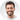#### RodrigoStecanella

September 21st, 2017

## Posts you might like...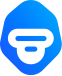## Text Analysis with Machine Learning

Turn tweets, emails, documents, webpages and more into actionable data. Automate business processes and save hours of manual data processing.